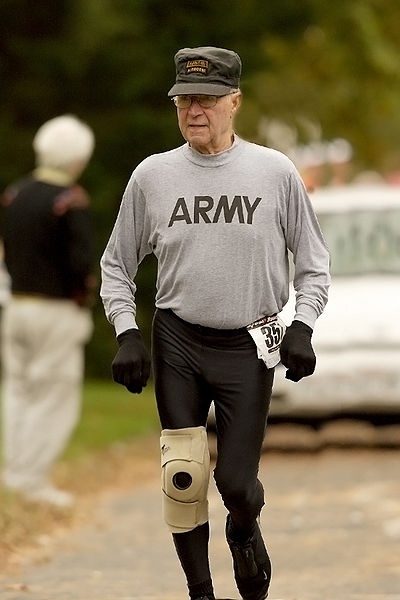Would Tripping Lose Boris The Race?Alfred and Boris have a $70$ km race. Alfred runs at a constant velocity of $7$ km h$^{-1}$, while Boris runs at a constant velocity of 10 km h$^{-1}$. However, during the contest, Boris trips and subsequently runs at a constant velocity of 5 km h$^{-1}$ as his knee hurts.

We do not know when Boris tripped. If we assume that the position that Boris trips is random, then the probability that Alfred won the race can be expressed as $\frac ab$ where $a$ and $b$ are positive co-prime integers. Find the value of $a+b$

×